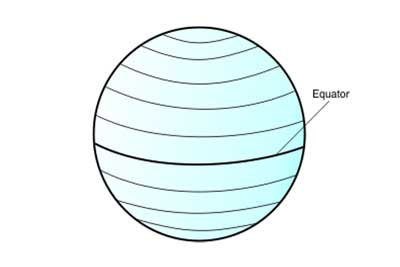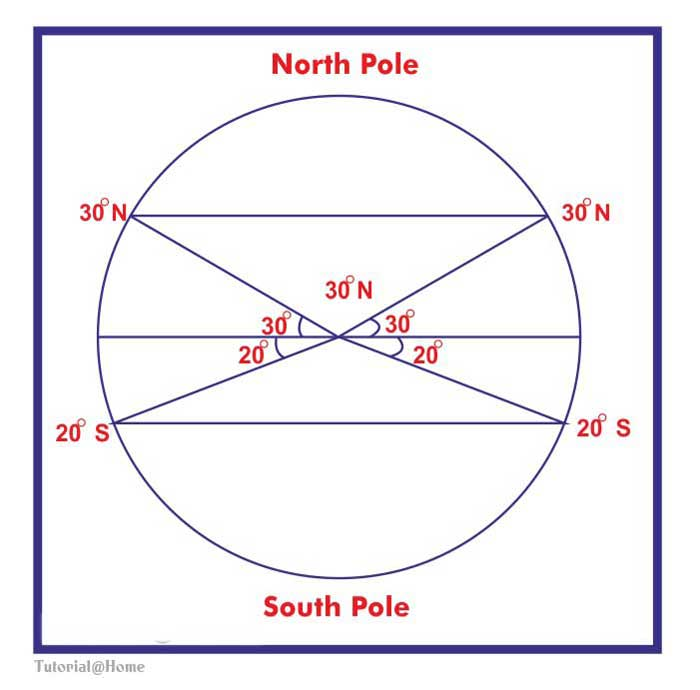Latitudes - Physical Geography
23-09-2018    323 timesThe angular distance of a place, north or south of the Equator, is called the ‘Latitude’ of that place. Since the Equator is at the 0º latitude and is the exact midpoint between the two Poles, it is the natural starting point for measuring the latitude or distance, north or south. The shortest route from the Equator to the Poles would cover one quarter of a circle or 90º. So the angular distance of each Pole from the Equator is 90º.

Between the Equator and the Poles, 90 lines parallel to the Equator can be drawn at an interval of 1º. Since these lines are parallel to the Equator, they are named ‘Parallels of Latitude’.  Each parallel forms a circle as it goes round the globe. The circles get smaller away from the Equator, till at the Poles they become merely points. The North and South Poles are called 90ºN and 90ºS respectively, since the angle between the Equator and any Pole is 90º. A place lying to the south of the Equator has a ‘south latitude’ while a place lying north of the Equator has a ‘north latitude’. Similarly, 24ºS latitude makes an angle of 24º with the Equator in the southern hemisphere while 60ºN latitude makes an angle of 60º in the northern hemisphere. Thus, if latitudes are drawn at 1º intervals, the total number of latitudes, including the Equator, will be 90+1+90 = 181. The latitude of a place is, therefore, the angular distance of a point, north or south of the Equator. It is measured in degrees from the centre of the Earth. On the other hand, the parallels of latitudes are the lines joining places having the same latitude.However, degrees are too large to measure precise distances. To accurately determine the exact position of a particular place on the globe, each degree of latitude is therefore, divided into 60 minutes and one minute is further divided into 60 seconds. Due to Polar flattening, the linear distance of a degree of latitude is slightly longer at the Poles than that at the Equator. However, the average distance of one degree is equal to about 111 km, and it can be obtained by dividing the Earth’s circumference (about 40,000 km) by 360º. Thus, Kolkata (22º34’N) is at a distance of about 2,500 km from the Equator.

The latitudes, that are near the Poles, between 90º and 60º, are known as the ‘High Latitudes’. The Latitudes between 60º and 30º are the ‘Mid Latitudes’, while the Latitudes that are near or to the Equator, between 30º and 0º, are called the ‘Low Latitudes’.Query About the post